# T-SQL Regular expression: LIKE Operator and its use-cases.

Total: 2 Average: 5

A regular expression is a rule which defines how characters can appear in an expression. It’s a sequence of character or text which determines the search pattern. In SQL databases, selecting values based on regular expressions defined in the WHERE condition can be very useful. Following are a few use cases of how you can use regular expressions.

1. It can be used to identify the data that uses a combination such as a credit or debit card number, an email address, or a telephone number.
2. Find specific text pattern or apply a filter on text, numeric, or special character data.
3. It can be used to parse the data in ETL by creating rules for inbound and outbound traffic, finding patterns in code.

Unlike MySQL and Oracle, SQL Server database does not support built-in RegEx functions. However, SQL Server offers built-in functions to tackle such complex issues. Examples of such functions are LIKE, PATINDEX, CHARINDEX, SUBSTRING and REPLACE. We can combine these functions with others and create a sophisticated and more complex query. These queries are difficult to maintain and requires more time and effort to develop it if we are querying a large table than these queries can have a huge impact on performance.

In this article, I am going to explain LIKE operator which can be used for pattern matching. Moreover, I will demonstrate the different use cases where we can use LIKE operator to search for data from a table based on a specific pattern.

The LIKE operator uses a combination of matching expression and pattern. The LIKE operator supports the pattern of following valid wildcard characters.

Wildcard CharacterDescription
%A string of zero or more character. For example, Employee_Name %Nisarg% will populate the records that have Nisarg word anywhere in the string.
[ ]Any single character within a specified range. The character The characters within an opening square bracket ( [ ) and opening square bracket ( ] ) are used for the matching process.
[^]None of the single character within the specified range. WHERE Employee_Name LIKE 'Ni[^A]%' will populate the records starting with Ni and where the following letter isn't A.

In this article, I am going to demonstrate the following use cases of the LIKE operator.

## Prepare Demo Setup

First, create a demo table named “Patient_Addresses.” To do that, execute the following query:

Now, execute the following query to insert data in “Patient_Addresses”.

Once data is inserted, execute the following query to review the data.

Data should look like the following.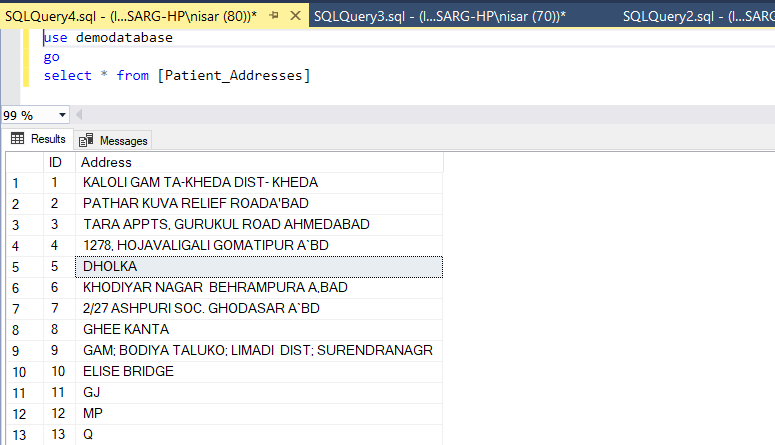Now let me explain the use cases.

Example 1:

For example, I want to populate only those rows which start with PA. To populate the data, we can use regex “[XY]%.“ To do that, execute the following query.

Following is the output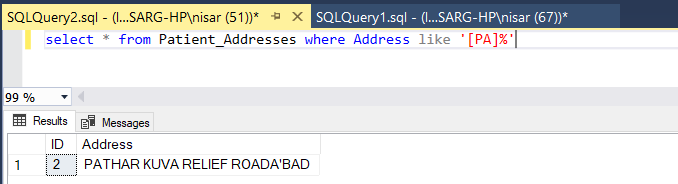As you can see in the above image, query retrieved only record where the value of the address column starts with “PA”

Example 2:

For example, I want to populate the only records which start with only two characters. The first character must be “E” and the second character must be “L.” To do that, execute the following query

Following is the output: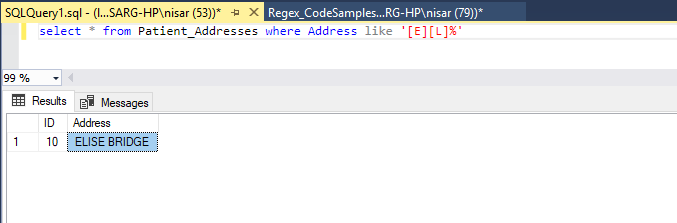As you can see from the image above, the query retrieved only record where the value of the address column that has “E” as the first character and “L” as the second character.

Example 3

For example, we want to retrieve only those rows which have two characters, and those characters should be between A to Z hence, the query should be written in the following structure:

Following is the output.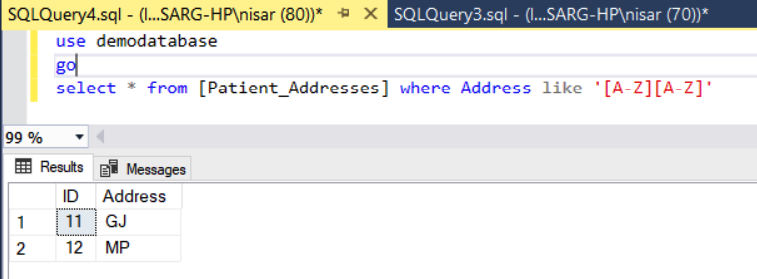As you can see from the image above,  the query returns the data, which has precisely two characters, and the value of both characters are between A and Z.

Example 4

Now we want to retrieve data, where the first character will be between K to P and the rest of the string, will be the same. To retrieve such format, use the following structure.

Following is the output: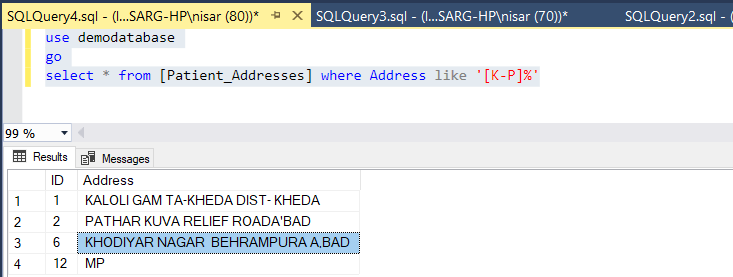Similarly, we can retrieve data where the last three characters will be “BAD”, and except those characters, the string will remain the same. To do that, execute the following query.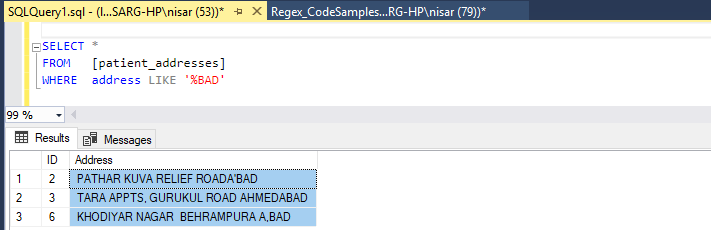Example 5

For example, we want to retrieve the list of addresses where the first character of the string must be between E and H, and the remaining string must remain the same. To do that we will use regex [X-Y]%. Execute the following query:

Following is the output.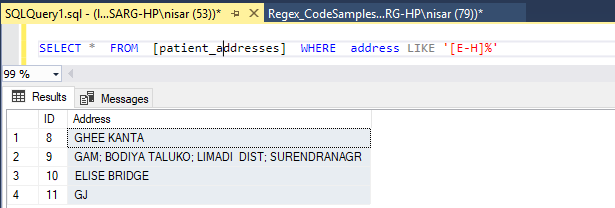Similarly, we can retrieve the list of addresses where the last character of the address column must be between A and C, and the remaining string must remain the same. To do that we will use regex %[X-Y]. Execute the following query

Following is the output.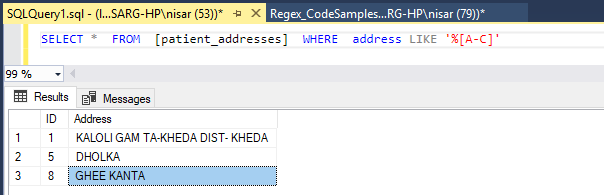Now let us see some complex examples.

Example 6

We want to populate the records from the address table where the last character must not be between B and D. To do that we will use regex %[^X-Y]. Execute the following query.

Following is the output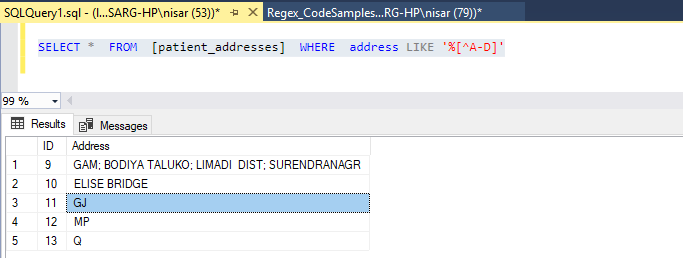Similarly, we want to retrieve the records where the first character of address should not be between A to Z. To do that we should use regex [^X-Y]% pattern. Execute the following query.

Following is the output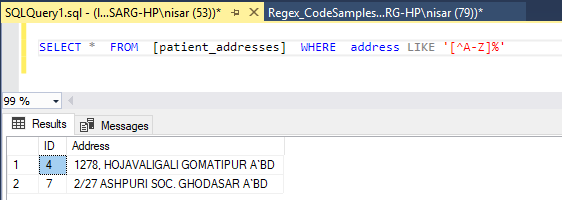## Find specific string pattern

Using Regular expression, we can find a specific text pattern. For example, I want to populate the records with the following patterns:

1. Any characters are allowed at first (first %),
2. The third character should be either I or S,
3. Forth and the fifth character will be SE. These characters are static.
4. The fifth character will be a space.
5. And then any character is allowed after that (last %).

To populate the record, execute the following query.

Following is the output.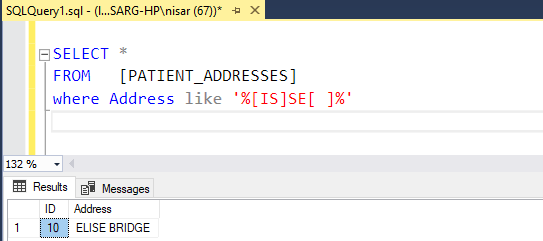## Summary